Question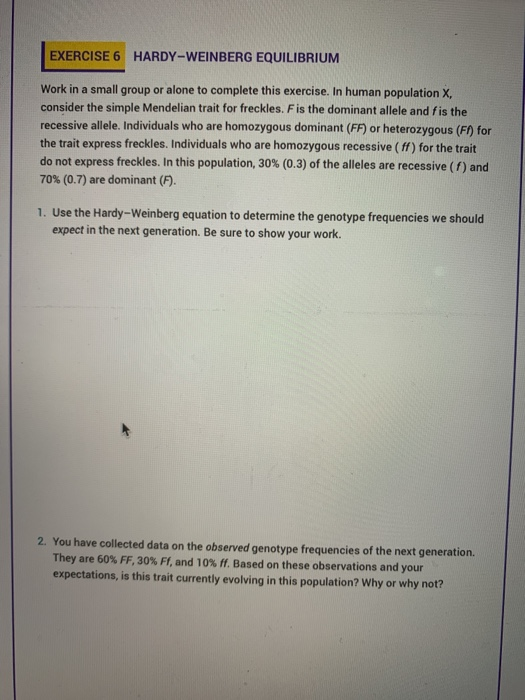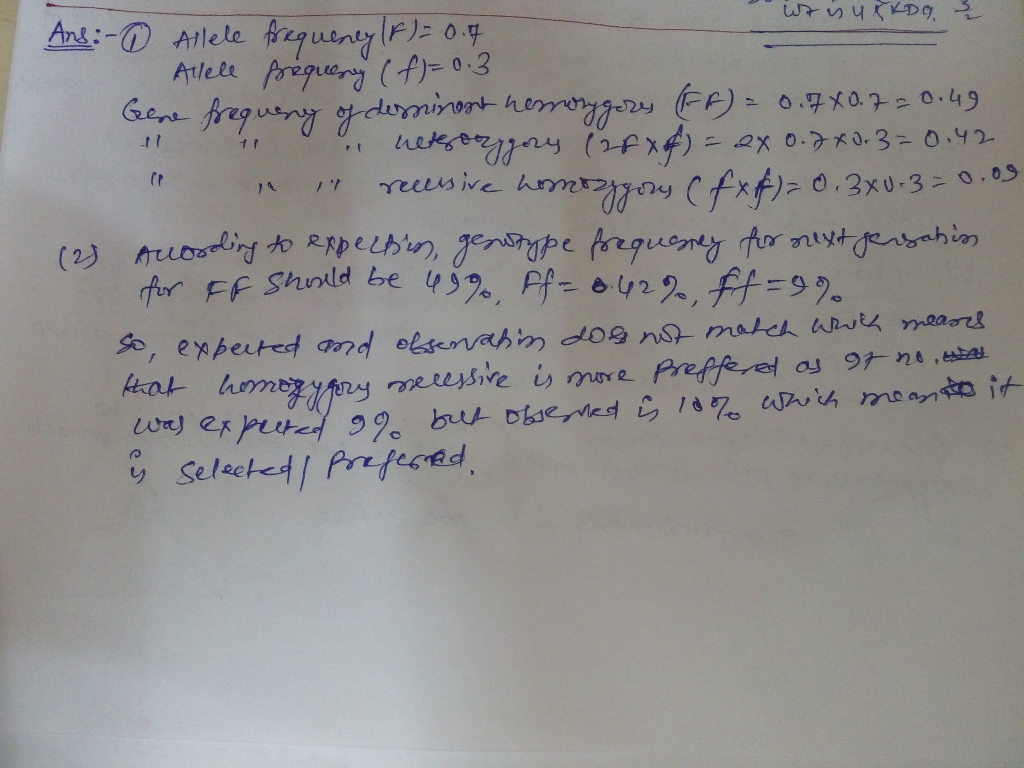Hope that's clear..thanks

#### Earn Coins

Coins can be redeemed for fabulous gifts.

Similar Homework Help Questions
• ### The Hardy-Weinberg principle and its equations predict that frequencies of alleles and genotypes remain constant from generation to generation in populations that are not evolving

.1. The Hardy-Weinberg principle and its equations predict that frequencies of alleles and genotypes remain constant from generation to generation in populations that are not evolving. What five conditions does this prediction assume to be true about such a population? a._______  b._______  c._______  d._______  e._______  2. Before beginning the activity, answer the following general Hardy-Weinberg problems for practice (assume that the population is at Hardy-Weinberg equilibrium).a. If the frequency of a recessive allele is 0.3, what is the frequency of the dominant...

• ### EXERCISE 1 CREATING PUNNETT SQUARES Work in a small group or alone to complete this exercise....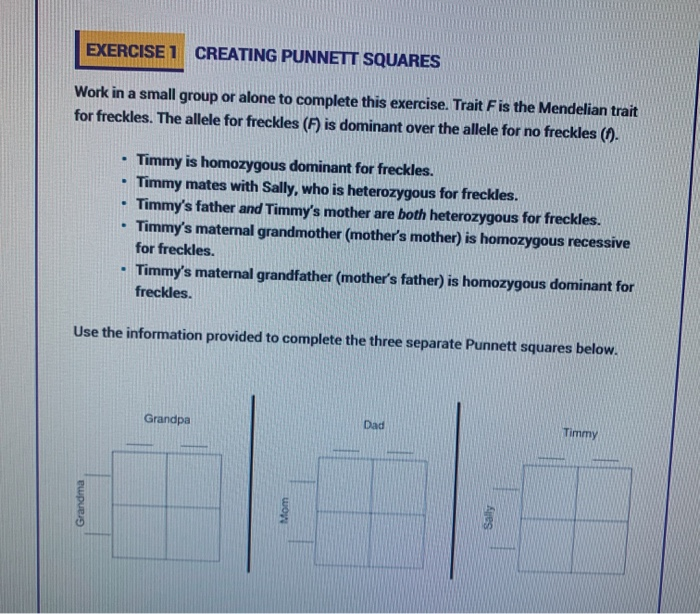EXERCISE 1 CREATING PUNNETT SQUARES Work in a small group or alone to complete this exercise. Trait F is the Mendelian trait for freckles. The allele for freckles (F) is dominant over the allele for no freckles (1) • Timmy is homozygous dominant for freckles. Timmy mates with Sally, who is heterozygous for freckles. Timmy's father and Timmy's mother are both heterozygous for freckles. Timmy's maternal grandmother mother's mother) is homozygous recessive for freckles. Timmy's maternal grandfather (mother's father) is...

• ### 2.3 Problem 3 The Hardy-Weinberg equation is useful for predicting the percent of a hu- man...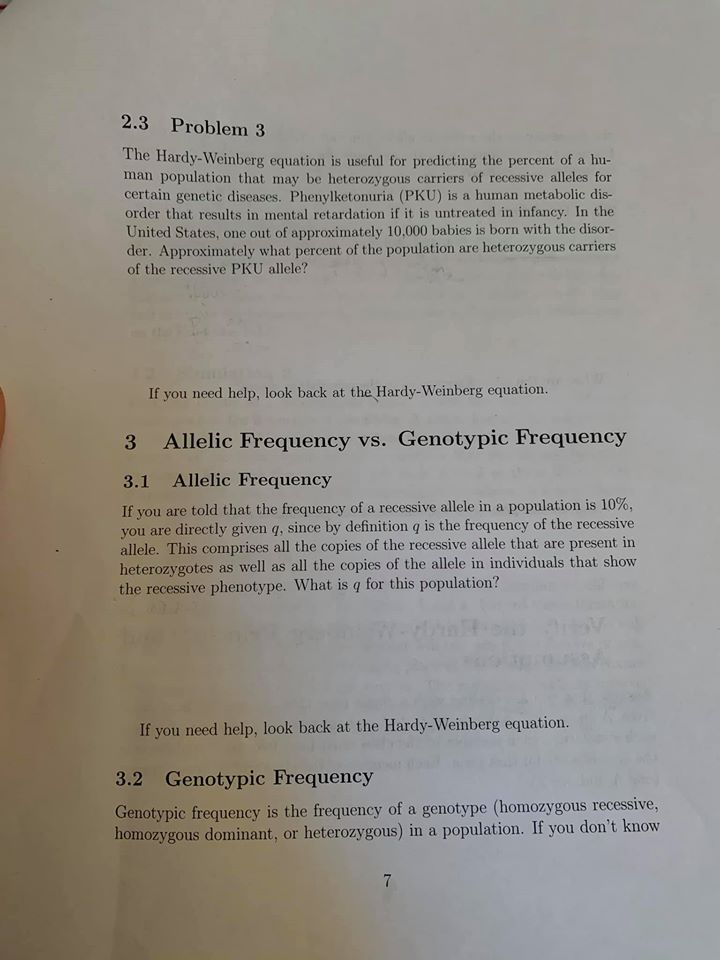2.3 Problem 3 The Hardy-Weinberg equation is useful for predicting the percent of a hu- man population that may be heterozygous carriers of recessive alleles for certain genetic diseases. Phenylketonuria (PKU) is a human metabolic dis- order that results in mental retardation if it is untreated in infancy. In the United States, one out of approximately 10.000 babies is born with the disor- der. Approximately what percent of the population are heterozygous carriers of the recessive PKU allele? If you...

• ### please click on the photo to see all of it The basic equations of Hardy-Weinberg Equilibrium...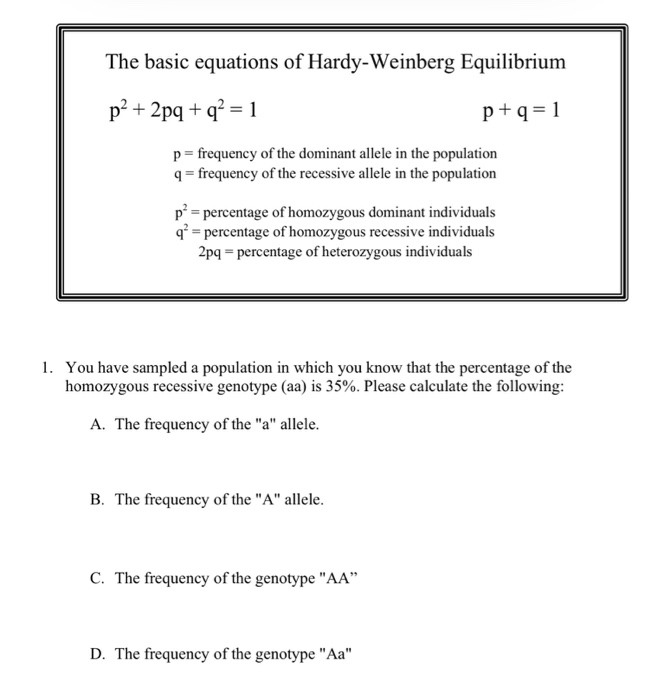please click on the photo to see all of it The basic equations of Hardy-Weinberg Equilibrium p² + 2pq + q2 = 1 p+q=1 p= frequency of the dominant allele in the population 9 = frequency of the recessive allele in the population př= percentage of homozygous dominant individuals q* = percentage of homozygous recessive individuals 2pq - percentage of heterozygous individuals 1. You have sampled a population in which you know that the percentage of the homozygous recessive genotype...

• ### Testing the goodness of fit between the data and the Hardy Weinberg equilibrium model generated expectations....

Testing the goodness of fit between the data and the Hardy Weinberg equilibrium model generated expectations. 4.1 In a species of bird, feather color is controlled by genes at a single locus, with the red feather allele dominant to the yellow feather allele. A population has 22 red and 14 yellow birds, with 9 of the red birds having a homozygous dominant genotype. Is this population in equilibrium? Calculate p and q from the number of individuals of each genotype:...

• ### Consider a locus of interest that has two alleles: A and a. A diploid individual carrying...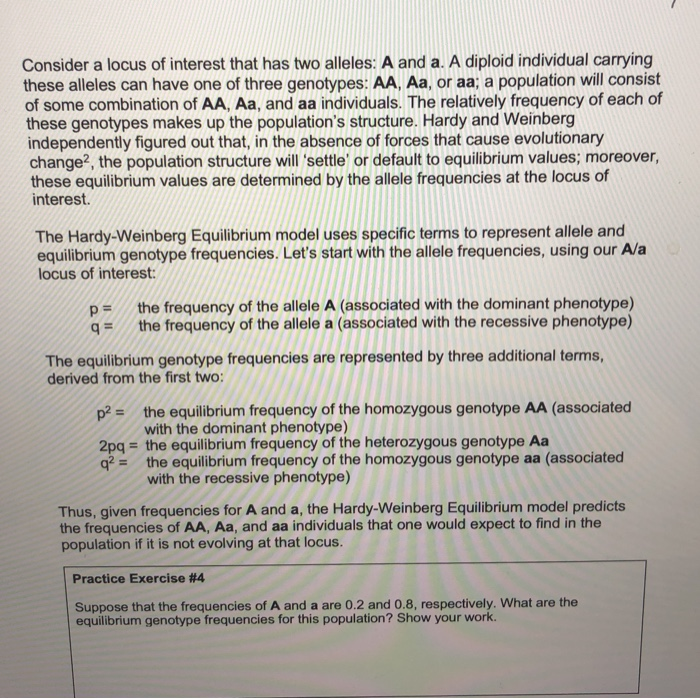Consider a locus of interest that has two alleles: A and a. A diploid individual carrying these alleles can have one of three genotypes: AA, Aa, or aa; a population will consist of some combination of AA, Aa, and aa individuals. The relatively frequency of each of these genotypes makes up the population's structure. Hardy and Weinberg independently figured out that, in the absence of forces that cause evolutionary change, the population structure will 'settle' or default to equilibrium values,...

• ### Use the Hardy-Weinberg equations (p2 + 2pq + q2 = 1 and p + q =...

Use the Hardy-Weinberg equations (p2 + 2pq + q2 = 1 and p + q = 1) to find the genotypes of the following hypothetical phenotypic information: a.       Within a particular population, you observe that 450 individuals have freckles (dominant, F) and 550 do not have freckles (recessive, f). b.      Homozygous dominant (frequency) ______(A)_________ c.       Heterozygous (frequency) ______(B)_____________ d.      Homozygous recessive (frequency) _______(C)____________

• ### reting Data: Hardy-Weinberg Equation 2 of 10 you use the Hardy Weinberg equation to answer questions...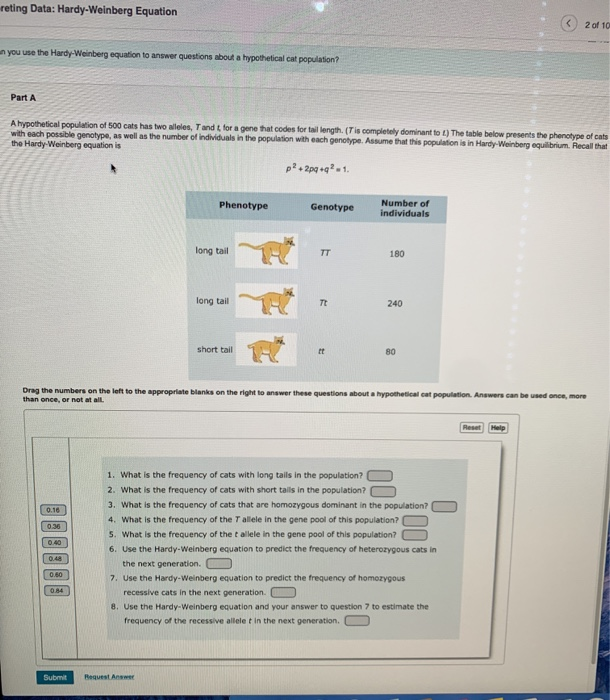reting Data: Hardy-Weinberg Equation 2 of 10 you use the Hardy Weinberg equation to answer questions about a hypotheticalcat population Part A A hypothetical population of 500 cats has two wees, Tandt for a gene that codes for tail length (Tis completely dominantot) The table below presents the phenotype of cats with each possible genotype, as well as the number of individuals in the population with each genotype. Assume that this population is in Hardy-Weinberg equilibrium Recall that the Hardy...

• ### Hardy-Weinberg Practice Problems: You need to list equations used and provide steps of problem solving. Providing...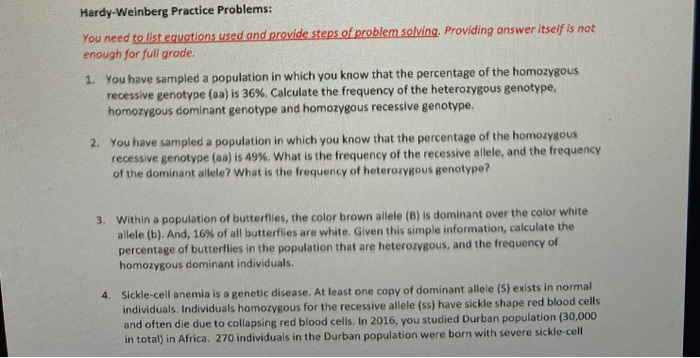Hardy-Weinberg Practice Problems: You need to list equations used and provide steps of problem solving. Providing answer itself is not enough for full grade. 1. You have sampled a population in which you know that the percentage of the homozygous recessive genotype (aa) is 36%. Calculate the frequency of the heterozygous genotype, homozygous dominant genotype and homozygous recessive genotype. 2. You have sampled a population in which you know that the percentage of the homozygous recessive genotype (aa) is 49%....

• ### (Hardy Weinberg Equation) In a population of rabbits, there are 423 A1A1, 1484 A2A2, and 1661...

(Hardy Weinberg Equation) In a population of rabbits, there are 423 A1A1, 1484 A2A2, and 1661 A1A2 individuals. If the enviornment changes so that the homozygous recessive genotype suffers a reduction of fitness where it's fitness is now 0.59 , but the other genotypes are unaffected, what will be the frequency of the dominant allele in the NEXT generation?